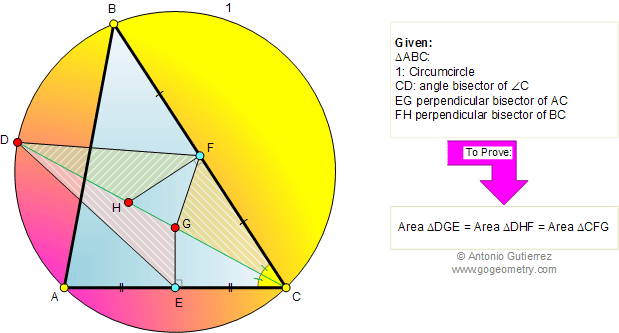Home Geometry Problems All Problems Puzzle 401-410 Perpendicular Bisector Area of a Triangle Solution/comment        By Antonio Gutierrez
 Problem 401. Area, Triangle, Angle bisector, Circumcircle, Perpendicular bisector, Congruence. Level: High School, College, SAT Prep. The figure shows a triangle ABC with the circumcircle 1. CD is the angle bisector of angle C. FH is the perpendicular bisector of BC and EG is the perpendicular bisector of AC. Prove that triangles DGE, DHF, and CFG have equal area.Recent Additions